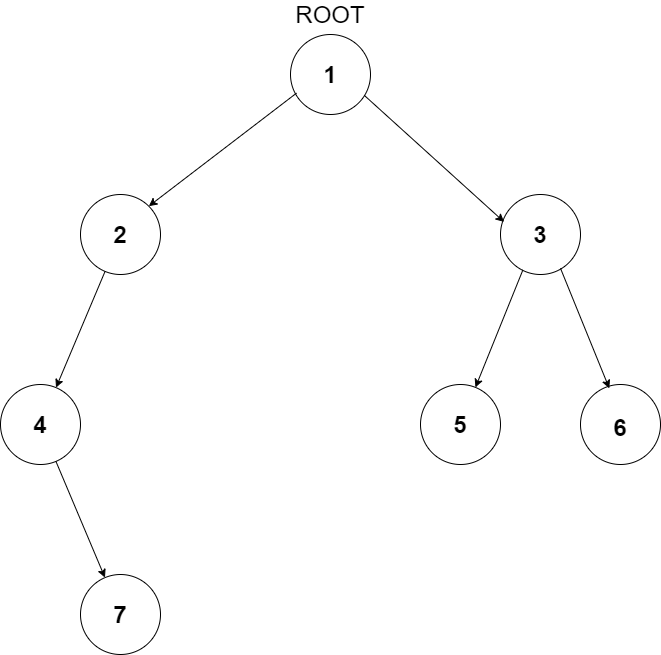0

Search In BST

Difficulty: EASY
Avg. time to solve
15 min
Success Rate
85%

Problem Statement

A binary search tree (BST) is a binary tree data structure that has the following properties.

1. The left subtree of a node contains only nodes with data less than the node’s data.
2. The right subtree of a node contains only nodes with data greater than the node’s data.
3. Both the left and right subtrees must also be binary search trees.
Note:
1.  It is guaranteed that all nodes have distinct data.
Input Format:
The first line contains a single integer ‘T’ representing the number of test cases.

The first line of each test case contains two positive integers ‘N and ‘X’, denoting the number of nodes in the BST and a given integer.

The second line contains the elements of the tree in the level order form separated by a single space. If any node does not have a left or right child, we take -1 in its place. Refer to the example below for further clarification.

For example, the input for the tree depicted in the below image would be :1
2 3
4 -1 5 6
-1 7 -1 -1 -1 -1
-1 -1

Explanation :
Level 1 :
The root node of the tree is 1

Level 2 :
Left child of 1 = 2
Right child of 1 = 3

Level 3 :
Left child of 2 = 4
Right child of 2 = null (-1)
Left child of 3 = 5
Right child of 3 = 6

Level 4 :
Left child of 4 = null (-1)
Right child of 4 = 7
Left child of 5 = null (-1)
Right child of 5 = null (-1)
Left child of 6 = null (-1)
Right child of 6 = null (-1)

Level 5 :
Left child of 7 = null (-1)
Right child of 7 = null (-1)

The first not-null node (of the previous level) is treated as the parent of the first two nodes of the current level. The second not-null node (of the previous level) is treated as the parent node for the next two nodes of the current level and so on.

The input ends when all nodes at the last level are null (-1).
Note:
The above format was just to provide clarity on how the input is formed for a given tree.

The sequence will be put together in a single line separated by a single space. Hence, for the above-depicted tree, the input will be given as:

1 2 3 4 -1 5 6 -1 7 -1 -1 -1 -1 -1 -1
Output Format:
For each test case, print “True” if there is a node in BST having data equals to ‘X’, otherwise print “False”.

Output for every test case will be printed in a separate line.
Note:
You don’t need to print anything; It has already been taken care of. Just implement the function.
Constraints:
1 <= T <= 50
1 <= N <= 10000
0 <= X <= 10^9
0 <= DATA <=10^9

Where ‘T’ is the number of test cases, ‘N’ is the number of nodes in the BST, ‘X’ is a given integer and ‘DATA’ is the data contained in each node of BST.

Time limit: 1 sec
Sample Input 1:
2
1 1
1 -1 -1
7 8
1 2 3 4 -1 5 6 -1 7 -1 -1 -1 -1 -1 -1
True
False
Explanation For Sample Input 1:
In the first test case, there is only one node and it has data 1, thus we should print ‘True’.

In the second test case, there is no node having data 8. See the problem statement for the picture of this BST.
Sample Input 2:
2
4 1
3 1 5 -1 2 -1 -1 -1 -1
4 6
3 1 5 -1 2 -1 -1 -1 -1
True
False
Explanation For Sample Input 1:
In the first test case, there is left node and it has data 1, thus we should print ‘True’.

In the second test case, there is no node having data 6. thus we should print ‘False’.Console